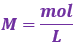# Problem: Calculate the pH after 0.020 mole of NaOH is added to 1.00 L of each of the following solutions:a. pure H2Ob. a mixture containing 0.100 M HC3H5O2 and 0.100 M NaC3H5O2

###### FREE Expert Solution

We are asked to calculate the pH after 0.020 mole of NaOH is added to 1.00 L of each of the following solutions

a. pure H2O

pH will depend on NaOH  (strong base)

Calculate pOH:

pOH = 1.70

Calculate pH :

pH = 12.30

The resulting solution after the titration has a pH of 12.30.

b. a mixture containing 0.100 M HC3H5O2 and 0.100 M NaC3H5O2

Step 1. Calculate the initial amounts of HC3H5O2 in moles before the reaction happens.

molarity (volume) → moles

Recall:*convert volumes from mL to L → 1 mL = 10-3 L

1.00 L of 0.100 M HC3H5O2

mol HClO = 0.100 mol HClO

1.00 L of 0.100 M  NaC3H5O2

mol  NaC3H5O2 = 0.100 mol  NaC3H5O2

Step 2. Write the chemical equation for the reaction between HC3H5O2 and NaOH.

Reaction:

HC3H5O2(aq)    +   NaOH(aq)               C3H5O2(aq)       +       H2O(aq)
(weak acid)          (base)                    (conjugate base)     (conjugate acid)

93% (318 ratings)###### Problem Details

Calculate the pH after 0.020 mole of NaOH is added to 1.00 L of each of the following solutions:

a. pure H2O
b. a mixture containing 0.100 M HC3H5O2 and 0.100 M NaC3H5O2